﻿ Simplification of Combustion AnalysisPublications are Open
Access in this journal
Article Versions
Export Article
• Normal Style
• MLA Style
• APA Style
• Chicago Style
Research Article
Open Access Peer-reviewed

### Simplification of Combustion Analysis

Balakrishnan Viswanathan , Mohamed Shajahan Gulam Razul
World Journal of Chemical Education. 2020, 8(2), 100-103. DOI: 10.12691/wjce-8-2-6
Received April 11, 2020; Revised May 13, 2020; Accepted May 20, 2020

### Abstract

Combustion analysis is typically taught within the context of molecular stoichiometry, in particular the study of empirical formula. This approach is rich in the history of chemistry and also relevant to current laboratory practice. However, the complexity inherent in the traditional approach is not suitable for beginning learners; what should be a rich context for exploring chemical connections has in effect been reduced to an algorithm to be memorized. It is proposed that combustion analysis be taught within the context of limiting reagents, as this represents an ideal situation (one reactant in limited quantity and the other in infinite quantity). Moving the pedagogical position of this topic from molecular stoichiometry to reaction stoichiometry reduces the complexity of the problem from four steps to two. More importantly, the proposed method makes it possible to harness the knowledge that students have acquired earlier in the course to solve a problem in a new context. In the new approach, students match coefficients (computed on the reactant side with unknown on the product side) to obtain the composition of the substance being combusted. It is expected that the connection to prior knowledge and acquired skills will help students achieve improved success when solving problems of this nature.

### 1. Introduction

The typical first-year program generally contains a section on combustion analysis within the discourse on stoichiometry. This section provides an example of one use of stoichiometry: to determine the composition of an unknown material. Combustion of a general unknown organic compound in excess oxygen can be expressed chemically as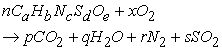(1)

Students are generally provided with the mass of substance burned, masses of products evolved, and the molar mass of the initial substance. Sometimes, the amount of one of the elements is determined by mass difference. The composition is determined by 1, 2

• Determining the moles of product (p, q, r, s) produced by combustion,

• Computing the moles of each element present in the reactant that was combusted,

• Reducing the moles of elements to the lowest whole number ratio to yield the empirical formula, and

• Scaling the empirical formula by the ratio of molecular molar mass and empirical formula mass to yield the molecular formula.

This traditional algorithm represents a historical approach to the problem of determining the formula of an unknown (pure) substance. This approach also directly applies several concepts explicitly. The concepts applied are (sequentially):

• The definition of molar mass

• Law of Conservation of Mass

• Principle of non-transmutability of elements

• The definition of empirical formula

• The relationship between empirical formula and molecular formula

It is seen that each of the concepts listed above is applied directly to the steps of the algorithm; each step involves one concept. Therefore, effective reproduction of the algorithm requires students to recall the necessary concepts (or their mathematical representations). Rote memorization of an algorithm reduces the ability (and motivation) of students to tackle problems conceptually 3; hence, in order to foster conceptual understanding students should not be encouraged to memorize complex algorithms.

The traditional approach is steeped in rich history and is likely translatable to professional laboratory practice. However, the approach and its nuances are unlikely to resonate with students who are new to the concepts of stoichiometry because of the large number of associations involved and the importance of the sequence. Effective learning requires “productive intermediate understandings” to support the conceptualization of chemical concepts. 4 Therefore, reducing the number of new associations during the exercise is an imperative for successful learning.

With the goal of improving students’ conceptual understanding, a new approach is proposed for presenting combustion analysis. The proposed method harnesses students’ prior knowledge to simplify the algorithm presented above.

### 2. Alternate Approach

Expecting students to remember to apply a large number of concepts in sequence reduces the exercise to an algorithm to be memorized, wherein students may not connect the steps to the concepts, leading to the material being forgotten rapidly 5. It is possible to reduce the number of concepts required to solve the problem. Students are generally adept at balancing equations by this stage in their coursework. Equation 1 above can be facilely balanced by first-year undergraduates symbolically to yield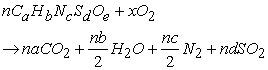(2)

Comparing the coefficients on the right-hand sides of Equations 1 and 2 yields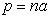(3a)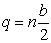(3b)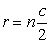(3c)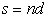(3d)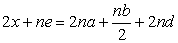(3e)

Since students are expected to be able to compute the number of moles of a substance given its amount and molar mass, they are able to compute n (this skill was used to compute the coefficients p, q, r, and s). Rearranging Equations 3a-d directly yields the subscripts in the original molecule, resulting in the molecular formula.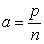(4a)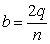(4b)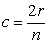(4c)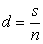(4d)

The amount of oxygen consumed in the reaction is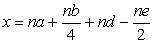(4e)

The process of determining the molecular formula is now simplified from four steps to two.

• Computing the numbers of moles of the unknown molecule and its combustion products, and

• Dividing the numbers of moles of products by the number of moles of the unknown, and multiplying by the number of atoms of the element in the product.

The empirical formula - if required - can be obtained post hoc by reducing the elemental subscripts to the lowest whole number ratio. The concepts that are required to solve this problem using this method are:

• The definition of molar mass (concept)

• Balancing of chemical reactions (skill)

○ Law of Conservation of Mass

○ Principle of non-transmutability of elements

• The relationship between empirical formula and molecular formula (concept).

Here, two of the important concepts have become implicit: mass conservation and non-transmutability are foundational to the balancing of chemical equations. Students now only apply two concepts (and one skill) in three steps, a much easier approach to solving the problem. By reducing the problem to one in which only two concepts need to be applied, these two concepts (definition of molar mass and number conservation) can be committed to long-term memory (through repetition) 6. Reducing the number of steps also reduces the complexity of the problem, thereby making it easier to understand and replicate. This allows the students to develop the skill of determining the molecular formula by performing combustion analysis.

The only new concept in the above list is the relationship between empirical formula and molecular formula. The rest of the problem can be framed as a basic problem in stoichiometry, no different from the concept of limiting reagents. Hence, limiting reagents is the context 7 for determining the molecular formula of an unknown organic compound by combustion analysis. Combustion analysis also provides another opportunity for practicing limiting reagents; in this sense combustion analysis is the application context for limiting reagents. Framing combustion analysis in terms of limiting reagents allows students to understand the latter concept through practice of an ideal system (oxygen is provided in unlimited quantities). Therefore, combustion analysis becomes an application of the concept of limiting reagents.

### 3. Examples

To aid educators, the proposed method is demonstrated (and compared with the traditional method) by means of some simple textbook examples. The examples presented below are taken verbatim from Ref. 1 and the solutions are generated and explained by the authors of the current submissions. All numbers are reported to the correct number of significant digits.

3.1. Example 1

Menthol, the substance we can smell in mentholated cough drops, is composed of C, H, and O. A 0.1005 g sample of menthol is combusted, producing 0.2829 g of CO2 and 0.1159 g of H2O. What is the empirical formula for menthol? If menthol has a molar mass of 156 g/mol, what is its molecular formula?

Step 1: Determine the amounts of CO2 and H2O produced

The amounts of CO2 and H2O produced are obtained by dividing the masses produced by their corresponding molar masses (CO2: 44.0095 g/mol, and H2O: 18.0153 g/mol). Hence, 6.428 mmol CO2 and 6.433 mmol H2O are produced in the experiment.

Step 2: Compute the amounts of C, H, and O in menthol

Each mole of CO2 contains one mole of carbon atoms. Hence, the amount of C atoms in the menthol sample is equal to the amount of CO2 produced upon combustion, or 6.428 mmol C atoms were present in the initial sample.

Each mole of H2O contains two moles of hydrogen atoms. Hence, the amount of H atoms in the menthol sample is equal to twice the amount of H2O produced upon combustion, or 12.866 mmol H atoms were present in the initial sample.

The amount of O in the sample is obtained by difference. The masses of C and H atoms are 0.0772 g and 0.01297 g, respectively. The mass of O atoms in menthol is then 0.01033 g. This is 0.644 mmol of O atoms.

Step 3: Determine the whole number ratio of C, H, and O

Dividing by the smallest amount yields the whole number ratio, which is C: H: O = 10: 20: 1. The empirical formula of menthol is then C10H20O1.

Step 4: Scale the empirical formula by the ratio of molar mass to empirical formula mass.

The empirical formula mass of C10H20O1 (obtained above) is 156 g/mol. The molar mass is given to be 156 g/mol. The ratio of the two yields 1. Hence, the molecular formula of menthol is C10H20O1.

3.1.2. Proposed Method

Step 1: Compute the amounts of menthol, CO2, and H2O

The amount of menthol is 0.644 mol; 6.428 mmol CO2 and 6.433 mmol H2O are produced in the experiment.

Step 2: Balance the combustion equation

The balanced combustion equation is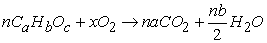(5)

which can be written as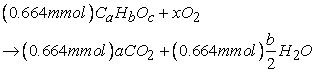(6)

Since (0.644 mmol)a = 6.428 mmol and (0.644 mol)b/2 = 6.433 mmol, the values of a and b are 9.98 and 20.0, respectively. The contributions of C atoms and H atoms to the molar mass of the molecule are 120 g/mol and 20.2 g/mol, respectively. By difference, O atoms contribute 16 g/mol, or c = 1.0. Hence, the molecular formula of menthol is C10H20O1 (rounded to the nearest whole numbers). The amount of oxygen gas consumed (x) is 9.34 mmol.

Step 3: Determine the empirical formula by reducing the molecular formula

The empirical formula is the smallest whole number ratio of elements in the molecular formula. In this case, it is not possible to reduce the formula further (the smallest number of atoms of an element is 1). Hence, the empirical formula of menthol is C10H20O1.

3.2. Example 2

Nicotine, a component of tobacco, is composed of C, H, and N. A 5.250 mg sample of nicotine was combusted, producing 14.242 mg of CO2 and 4.083 mg of H2O. What is the empirical formula of nicotine? If nicotine has a molar mass of 160 ± 5 g/mol, what is its molecular formula?

Step 1: Determine the amounts of CO2 and H2O produced

The amounts of CO2 and H2O produced are obtained by dividing the masses produced by their corresponding molar masses (CO2: 44.0095 g/mol, and H2O: 18.0153 g/mol). Hence, 0.32361 mmol CO2 and 0.2266 mmol H2O are produced in the experiment.

Step 2: Compute the amounts of C, H, and N in nicotine

Each mole of CO2 contains one mole of carbon atoms. Hence, the amount of C atoms in the menthol sample is equal to the amount of CO2 produced upon combustion, or 0.32361 mmol C atoms were present in the initial sample.

Each mole of H2O contains two moles of hydrogen atoms. Hence, the amount of H atoms in the menthol sample is equal to twice the amount of H2O produced upon combustion, or 0.2266 mmol H atoms were present in the initial sample.

The amount of N in the sample is obtained by difference. The masses of C and H atoms are 3.8868 g and 0.4568 g, respectively. The mass of N atoms in nicotine is then 0.01033 g. This is 0.0647 mmol of N atoms.

Step 3: Determine the whole number ratio of C, H, and N

Dividing by the smallest amount yields the whole number ratio, which is C : H : N = 5 : 7 : 1. The empirical formula is then C5H7N1.

Step 4: Scale the empirical formula by the ratio of molar mass to empirical formula mass.

The empirical formula mass of C5H7O1 (obtained above) is 81.1 g/mol. The molar mass is given to be 160 ± 5 g/mol. The ratio of the two yields 2 (rounded to the nearest whole number). Hence, the molecular formula is C10H14N2.

3.2.2. Proposed Method

Step 1: Compute the amounts of nicotine, CO2, and H2O

The amount of nicotine is 0.03281 mmol; 0.32361 mmol CO2 and 0.2266 mmol H2O are produced by combustion.

Step 2: Balance the combustion equation

The balanced combustion equation is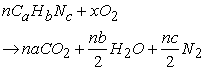(7)

which can be written as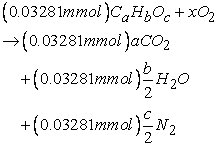(8)

Since (0.03281 mmol) a = 0.32361 mmol and (0.03281 mol) b/2 = 0.2266 mmol, the values of a and b are 9.863 and 13.81, respectively. The contributions of C atoms and H atoms to the molar mass of the molecule are 118.5 g/mol and 13.92 g/mol, respectively. By difference, N atoms contribute 28 g/mol, or c = 2.0. Hence, the molecular formula is C10H14N2 (rounded to the nearest whole numbers). The amount of oxygen gas consumed (x) is 0.4429 mmol.

Step 3: Determine the empirical formula by reducing the molecular formula

The empirical formula is the smallest whole number ratio of elements in the molecular formula. Hence, the empirical formula is C5H7N1.

### 4. Conclusion

This communication presents an alternate approach to presenting and solving combustion analysis problems to determine the molecular formula by utilizing students’ prior knowledge. The traditional four-step algorithm is simplified to a two-step method by treating the problem as an ideal limiting reagent problem (one of the two reagents is in infinite excess). Therefore, simple reaction balancing leads directly to determination of the molecular formula of the unknown compound. This section can then be taught as an application of limiting reagents instead of as the application of first-principle stoichiometric concepts to a real-world problem (taught prior to reaction balancing 1, 8); this is because the traditional mode teaches oxidation analysis as a concept related to mass composition. The traditional approach is valuable when the molar mass of the compound is not known, i.e., only the empirical formula is desired (and accessible). However, when the molar mass is known, the current method - invoking limiting reagents - provides a more direct means of obtaining the molecular formula. It is expected that teaching combustion analysis as a limiting reagent problem would improve understanding of limiting reagents - by providing a stepwise approach to the concept - while simplifying the algorithm (for oxidation analysis) and permitting the students to achieve educational success.

### Acknowledgements

This approach was motivated by similar (unsuccessful) approaches attempted by a section of BV’s first-year class under testing conditions.

### References

  T.L. Brown, H.E. LeMay Jr., B.E. Bursten, C.J. Murphy, P.M. Woodward, M.W. Stoltzfus, M.W. Lufaso, Chemistry: The Central Science, 14th ed., Pearson, New York, New York, 2018. In article  I.D. Reingold, A Concrete Analogy for Combustion Analysis Problems, J. Chem. Educ. 72 (1995) 222-223. In article View Article  E. Yuriev, S. Naidu, L.S. Schembri, J.L. Short, Scaffolding the development of problem-solving skills in chemistry: guiding novice students out of dead ends and false starts, Chem. Educ. Res. Pract. 18 (2017) 486-504. In article View Article  H. Sevian, V. Talanquer, Rethinking chemistry: a learning progression on chemical thinking, Chem. Educ. Res. Pract. 15 (2014) 10-23. In article View Article  A. Gilewski, E. Mallory, M. Sandoval, M. Litvak, L. Ye, Does linking help? Effects and student perceptions of a learner-centered assessment implemented in introductory chemistry, Chem. Educ. Res. Pract. 20 (2019) 399-411. In article View Article  J.R. Hartman, E.A. Nelson, “Do we need to memorize that?” or cognitive science for chemists, Found. Chem. 17 (2015) 263-274. In article View Article  M.M.W. Cheng, Students’ visualisation of chemical reactions - insights into the particle model and the atomic model, Chem. Educ. Res. Pract. 19 (2018) 227-239. In article View Article  M.S. Silberberg, Principles of General Chemistry, 2nd ed., McGraw-Hill Publishing Company, Dubuque, IA, 2010. In article

Published with license by Science and Education Publishing, Copyright © 2020 Balakrishnan Viswanathan and Mohamed Shajahan Gulam RazulThis work is licensed under a Creative Commons Attribution 4.0 International License. To view a copy of this license, visit http://creativecommons.org/licenses/by/4.0/